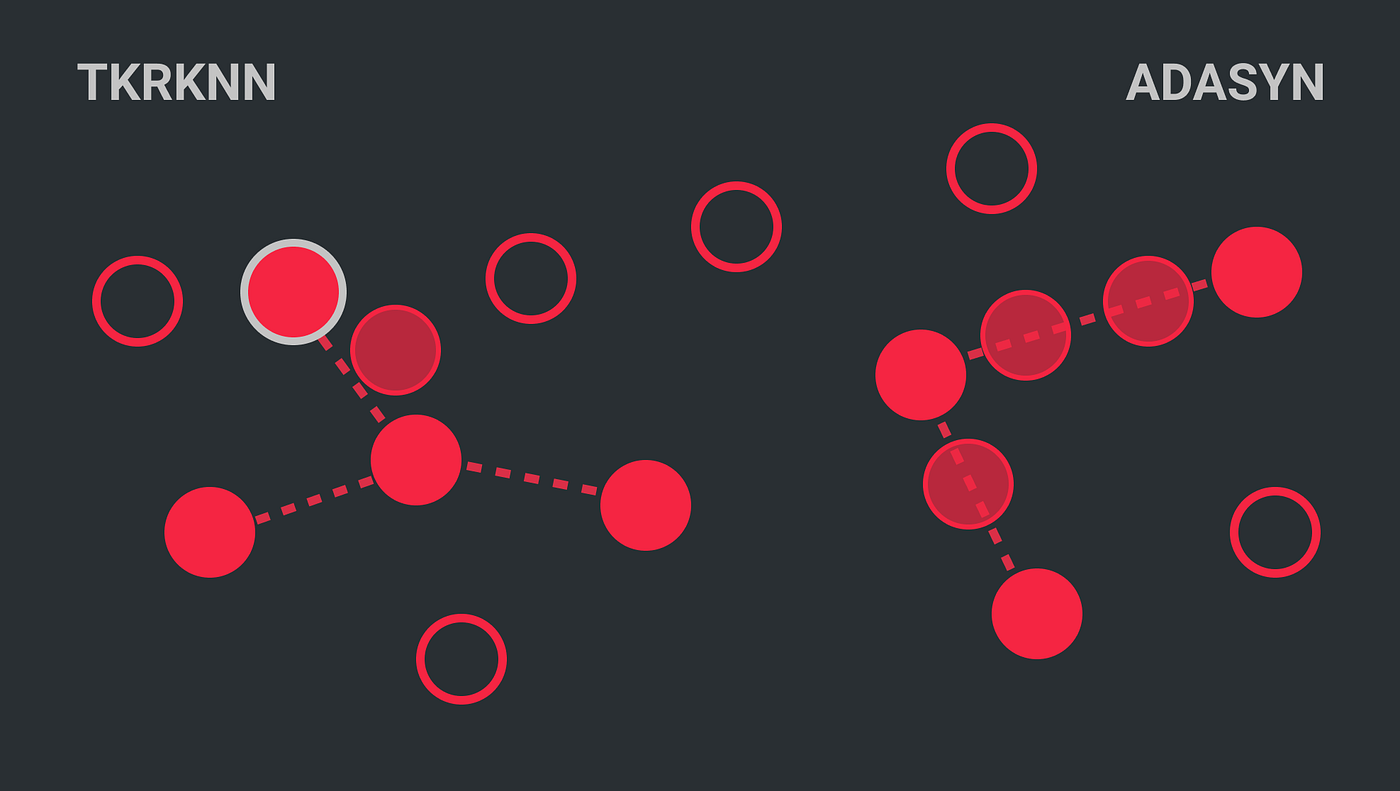# Generate new samples non-linearly using Crucio TKRKNN

Nowadays the majority of data sets in the industry are unbalanced. Meaning that a class has a higher frequency than others. Very often classifiers in such cases due to the unbalance of the data predict all samples as the most frequent class. To solve this problem we decided at Sigmoid to create a package that will have implemented all oversampling methods. We named it Crucio, and in this article, I will tell you about TKRKNN(Top-K Reversed KNN).

## How does TKRKNN work?

Firstly we start by finding the K-nearest neighbors for all samples of the minority class. Then, TKRKNN finds out the number of samples to generate which is equal to the number of minority samples subtracted from the number of majority samples.

Now, in order to generate every new sample, we are taking a random minority class sample and a random nearest neighbor. For every attribute of these vectors, we calculate the difference. This difference between these attributes is then multiplied by a random number — the gap, between 0 and 1, and added to the correspondent attribute to the initially selected minority class sample. This modified minority sample is then added to the synthetic data generated.

The difference between this algorithm and others is that the newly generated samples don’t stay anymore on the line between the samples used for their generation.Red points represent the minority class samples, the black ones the majority ones. The transparent ones are the new generated samples.

## Using Crucio ICOTE.

In case you didn’t install Crucio yet, then type in the terminal the following:

``````pip install crucio
``````

Now we can import the algorithm and create the TKRKNN balancer.

``````from crucio import TKRKNN

tkrknn = TKRKNN()
balanced_df = tkrknn.balance(df, 'target')
``````

TKRKNN constructor has only 3 parameters:

• binary_columns (list, default=None) : if set to then it will check if the listed columns are in the passed data frame then they will be transformed to binary ones after generating new points.
• seed (int, default = 42) : seed for random number generation.
• k (int, default = 5) : the number of nearest neighbors to find for every sample.

The balance function takes only 2 parameters:

• df (pd.DataFrame) : the pandas data frame that should be balanced.
• target (str) : the name of the column from the data frame that should be balanced.

Now let’s apply it to a data set. We will use it on the Pokemon dataset. The target column (Legendary) is unbalanced, only 8% is not legendary.
We recommend you, before applying any module from Crucio, to first split your data into a train and test data sets and balance only the train set. In such a way you will test the performance of the model only on natural data.
The results before balancing and after.

As we can see, TKRKNN is very conservative in generating new samples comparing with SMOTE or ICOTE especially, and other oversampling techniques. So, if you need more conservative generation of minority samples, go for TKRKNN.